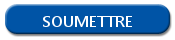# Write an excel file / xlsx with R

## Export an excel file in R with write.xlsx() function

Writing of Excel file isn't natively available in R. To do that, we can use the xlsx library and the function write.xlsx().

After installing the xlsx package, if necessary, you have to load the xlsx library and then use the write.xlsx() function. Here is an example to write an excel file:

```library(xlsx)
write.xlsx(x = myDataframe, file = "myFile.xlsx")```

Your data frame is exported in excel filetype to the root path of your work directory.

## Go further with the write.xlsx() function

Contrary to the .csv files, excel files have several advanced features to enrich tables. Take advantage of the write.xlsx() function and use the optional parameters to go further on the writing of Excel file in R.

```col.names = TRUE
row.names = TRUE
append = FALSE
showNA = TRUE```

For example, you can write excel files with several sheets overwriting your file and mentioning another name for the sheetName parameter.

```write.xlsx(x = myDataframe1, file = "myFile.xlsx", sheet = "Sheet1")
write.xlsx(x = myDataframe2, file = " myFile.xlsx", sheetName = "Sheet 2")```